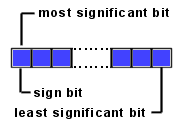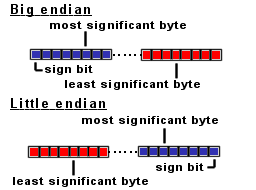# Java Quick Guide

This guide contains useful Java information.

## Java byte type.

Information
Byte types are so called signed two-complement numbers. The 2 complement scheme allows both positive and negative integers to be represented. The most significant bit of a two complement number is its sign bit. The sign bit is 1 for negative numbers and 0 for positive numbers and for 0.In Java the bytes are big-endian ordered, which means the most significant BYTE (where the sign bit is located) is always on the left side:Byte values are stored in 1 byte and contains positive or negative integer numbers.

The byte value range is: 28 = 256

Half of this number (256) are positive numbers ranging from: >=0 and <=127
For example:
0x00 = 0
0x01 = 1
0x7F = 127

The other half are negative numbers ranging from: >=-128 and <0
For example:
0xFF = -1
0x80 = -128

To convert negative decimal values into hexadecimal values:
value = 28 + (neg_dec_value)
value = 256 + (neg_dec_value)

For example:
neg_dec_value= -1
value = 256 + (neg_dec_value) = 256 + (-1) = 255
The new decimal value 255 equals 0xFF (Use your Windows calculator, to verify this).

During arithmetic operations the JVM always convert the byte value into an int.

 Type Min value Max value Byte -128 127